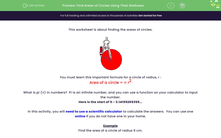# Find the Area of a Circle

In this worksheet, students will find the areas of circles given their radiuses.Key stage:  KS 3

Curriculum topic:   Geometry and Measures

Curriculum subtopic:   Solve Problems Involving Perimeters and Areas of 2D Shapes

Popular topics:   Area worksheets, Shape worksheets, Geometry worksheets

Difficulty level:#### Worksheet Overview

This activity is about finding the areas of circles.You need to learn this important formula for a circle of radius, r :

Area of a circle = π r2

What is pi (π) in numbers?  Pi is an infinite number, and you can use a function on your calculator to input the number.

Here is the start of it  3.14159265359...

In this activity, you will need to use a scientific calculator to calculate the answers.  You can use one online if you do not have one in your home.

Example

Find the area of a circle of radius 6 cm.

Area = π r2 =  π × 62 = π × 36 = 113.097335529

We need to give our answer to 1 decimal place

This means that if the second number after the decimal point is 5 or higher, then we round the number straight after the decimal point up. If the second number after the decimal point is less than 5, we leave the number after the decimal point the same.

Area = π r2 =  π × 62 = π × 36 = 113.1 cm²

Remember, in this activity, you need to use a scientific calculator to calculate pi correctly, you can access one of these online.

### What is EdPlace?

We're your National Curriculum aligned online education content provider helping each child succeed in English, maths and science from year 1 to GCSE. With an EdPlace account you’ll be able to track and measure progress, helping each child achieve their best. We build confidence and attainment by personalising each child’s learning at a level that suits them.

Get started